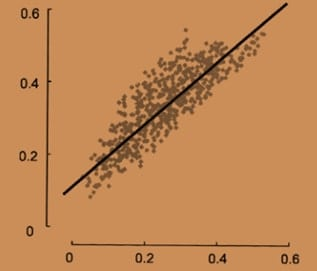# MCQ – 73837

### Question

The following scatter plot of 4 different samples shows the correlation between weight and height in the samples. All 4 samples have the same coefficient of correlation of 0.6 taken together, what will be the net correlation coefficient?A.

>0.6

B.

<0.6

C.

0.6

D.

Cannot be calculated